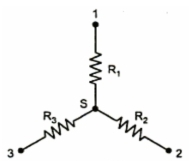Summary of Delta-Star and Star-Delta Transformation

Table 1 shows the equivalent Star and equivalent Delta resistances.Table .  1

Example 1: Convert the given Delta in the Fig. 1 into equivalent Star.Fig. 2
Solution : Its equivalent Star is as shown in the Fig. 2.
Where
R1 = (10 x 5 )/(5 +10 + 15) = 1.67 Ω
R2 = (15 x 10)/(5 +10 + 15) = 5 Ω
R3 = (5 + 15)/(5 + 10 + 15) = 2.5 ΩFig.  3

Example 2.: Find equivalent resistance between points A-B.Fig.  4

Solution : Redrawing the circuit,Fig. 4(aFig. 4(b

RAB = (21 x 14)/(21 + 14) = 8.4 Ω

Example 3 : Find equivalent resistance between point A-B.Fig.  5

Solution : Redraw the circuit,Fig.  5(aFig.  5(b

RAB = 8.4 Ω